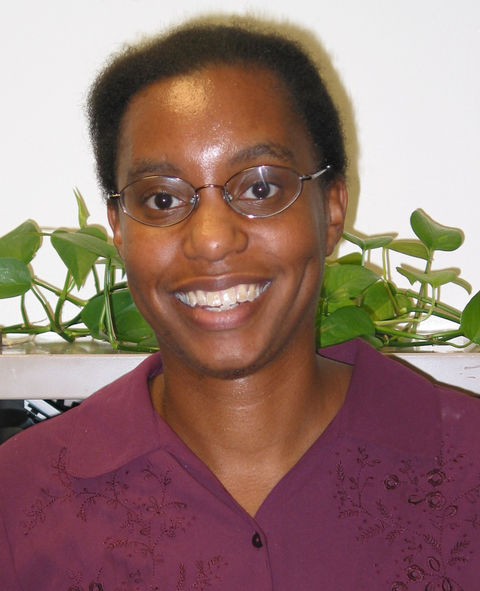# Anita Mareno, Ph.D.Associate Professor of Mathematical Sciences, School of Science, Engineering, and Technology
Olmsted Building, W255l
W255L OLMSTED BLDG
PENN STATE HBG
MIDDLETOWN, PA 17057

Dr. Anita Mareno graduated, summa cum laude in 1995, from Wellesley College, with a B.A. degree in mathematics and a minor in computer science. She was awarded a Ford Foundation Fellowship for graduate studies and obtained her Ph.D. degree in applied mathematics at the Center for Applied Mathematics in 2001, Cornell University, under the guidance of her advisor Prof. Timothy J. Healey (Dept. of Theoretical and Applied Mechanics). Shortly after graduating, Dr. Mareno was a VIGRE Assistant Professor in the Department of Mathematics at Cornell University where she had the opportunity to pursue her research interests in partial differential equations with some help and inspiration from those such as the late Lawrence Payne (Dept. of Mathematics). Dr. Mareno has been at Penn State Harrisburg since 2007. Some of her current and future plans at Penn State Harrisburg include developing and advising undergraduate research projects in mathematics and to conduct research knot theory and mechanics.

• Nonlinear elasticity, plate equations
• Dynamical systems and bifurcation theory
• Partial differential equations: maximum principles, existence & uniqueness, global continuation
• Calculus of variations

Anita Mareno, Lars Q. English. Snapback repellers in a coupled system of logistic maps. To be submitted.

Emma Heyd, Anita Mareno. Minimizers for hanging cables of unspecified length with variable endpoints. To be submitted.

Houssem Mhiri , Moyi Tian , Erin Wynne , Sean Jones , A. Mareno , L. Q. English. An Experimental Survey of Chaos and Symmetry breaking in coupled and driven logistic maps, submitted Eur. J. Phys. May 2019.

L.Q. English, A. Mareno. Symmetry breaking in symmetrically coupled logistic maps, Eur. J. Phys. 40 (2019), 024003.

A. Mareno. A maximum principle result for a general fourth order semilinear elliptic equation, Journal of Applied Mathematics and Physics 4 (2016), pp. 1682-1686.

C.P. Danet, A. Mareno. Maximum principles for a class of linear elliptic equation of even order, Mathematical Inequalities and Applications, 16 (2013) pp.809-822.

L.Q. English, A. Mareno. Orbits and Stability Analysis for the Coin Funnel Problem, accepted , American Journal of Physics, 80 (2012), pp. 996-1000.

A. Mareno. On maximum principles for m-metaharmonic equations, International Scholarly Research Network (ISRN) Mathematical Analysis 2012 (2012), pp. 1-9.

A. Mareno. Maximum principle results for a nonlinear equation from thin plate theory, Applied Mathematical Sciences 6 (2012), pp. 2307 – 2314.

A. Mareno. Maximum principles for some higher order semilinear elliptic equations, Glasgow Mathematical Journal 53 (2011), pp. 313-320.

A. Mareno. Maximum principles and bounds for a class of fourth order nonlinear elliptic equations, Journal of Mathematical Analysis and Applications 377 (2011), pp. 495-500.

A. Mareno. Integral bounds for von Karman’s equations, Zeitschrift für Angewandte Mathematik und Mechanik (Journal of Applied Mathematics and Mechanics) 90 (2010), pp. 509-513.

A. Mareno , L.Q. English. Catenary Stability for Variable-Length Cables, Wolfram Demonstrations Project, (2009).

A. Mareno, L.Q. English. Stability of the catenary shapes for hanging cables of unspecified lengths, Eur. J. Phys. 30 (2009), pp. 97-108.

A. Mareno. Maximum principles for a fourth order equation from thin plate theory, Journal of Mathematical Analysis and Applications 343 (2008), pp. 932-937.

Anita Mareno, Timothy J. Healey. Global Continuation in Second-Gradient Nonlinear Elasticity, SIAM Journal on Mathematical Analysis 38 (2006), pp. 103-115.

Anita Mareno. Uniqueness of Equilibrium Solutions in Second-Order Gradient Nonlinear Elasticity, J. Elasticity 74 (2004), pp. 99-107.

Publication List: C. Bu, R. Shull, Anita Mareno. Numerical Simulation of Blow-Up of a 2D Generalized Ginzburg -Landau Equation, Applied Math Letters 9 (1996), pp. 13-17.

Ph.D., Applied Mathematics (Cornell)

M.S., Applied Mathematics (Cornell)

B.A., Mathematics (summa cum laude) (Wellesley College)

Algebra and Trigonometry
Introduction to Statistics (without calculus)
Calculus I, II, III
Ordinary Differential Equations
200 and 400 Level Linear Algebra
Problem Solving in Mathematics (A 200 level course in mathematics content for prospective elementary school teachers)
Discrete Mathematics//Foundations of Mathematics
400 Level Foundations of Geometry
400 Level Partial Differential Equations and Fourier Series
400 Level Calculus of Variations
400 Level Abstract Algebra
400 Level Dynamical Systems course